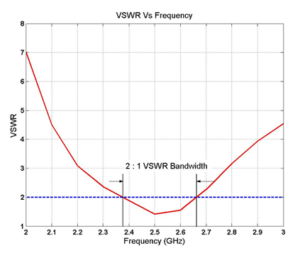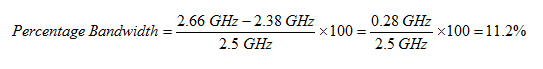# How do you specify the bandwidth of an antenna?

The bandwidth and frequency range of an antenna are related terms.

Bandwidth of an antenna is an important concept. The bandwidth of an antenna refers to the range of frequencies over which the antenna satisfies a particular parameter specification. The parameters generally specified are gain, radiation pattern, the VSWR etc. Most commonly, the VSWR is chosen as the parameter for bandwidth considerations and this bandwidth is called the impedance bandwidth. The lower and upper frequencies conforming to the desired VSWR set the frequency band over which the antenna meets the VSWR specification. A VSWR specification commonly adopted is a 2:1 VSWR, which means that the range of frequencies over which the VSWR is less than 2 is chosen as the bandwidth of operation.As you can see from the plot, the absolute bandwidth over which the antenna meets the 2:1 VSWR specification is 2.66 GHz – 2.38 GHz = 0.28 GHz = 280 MHz.

Another commonly used way to specify bandwidth is percentage bandwidth, which is explained in more detail in the theory section. It requires specifying the center frequency of operation, which in this case is 2.5 GHz. For this antenna, the percentage bandwidth is:Want more valuable information?